# How to use the YEAR Function in Excel

The YEAR function is a Date & Time function. It returns the year of the date which is an argument to the function.
Syntax:

=YEAR (serial number)

Serial number : A valid date in Excel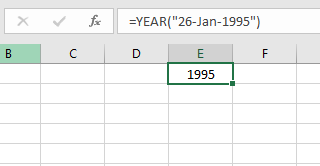Example:
Let’s understand this function using it in an example.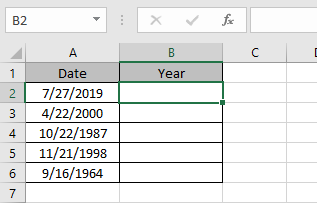Here we have a dates in column A. And we will use the YEAR function to get the year out in column B.

Use the formula:

=YEAR(A2)

A2 : A valid excel date as an argument to the function.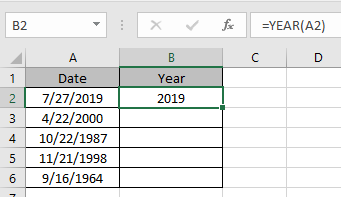As you can see from the above snapshot the year to date in Column B.
Copy the formula in other cells using the keyboard shortcut keys Ctrl + D.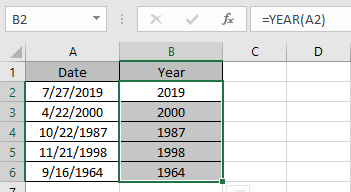The formula returns the Year from the Dates.
The function returns the #VALUE! error if text is given as an argument.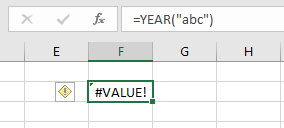Uses
Use the function with other functions like TODAY() to get the year of Today’s date. This returns a value which is dynamic. So the year will change as the Today function is dynamic.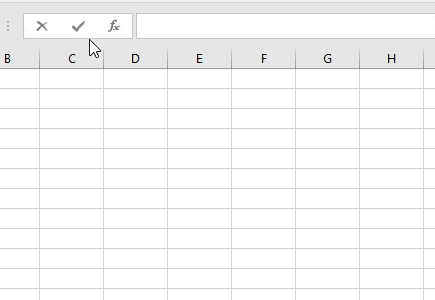Hope you understood how to use the YEAR function in Excel. Explore more articles on Excel function here. Please feel free to state your query or feedback for the above article.

Related Articles:

How to use the Excel TIME function

How to use the NOW Function in Excel

How to Use TODAY Function in Excel

How to use WEEKDAY Function in Excel

How to Use MONTH Function in Excel

Popular Articles:

50 Excel Shortcuts to Increase Your Productivity

How to use the VLOOKUP Function in Excel

How to use the COUNTIF in Excel 2016

How to use the SUMIF Function in Excel

Terms and Conditions of use

The applications/code on this site are distributed as is and without warranties or liability. In no event shall the owner of the copyrights, or the authors of the applications/code be liable for any loss of profit, any problems or any damage resulting from the use or evaluation of the applications/code.